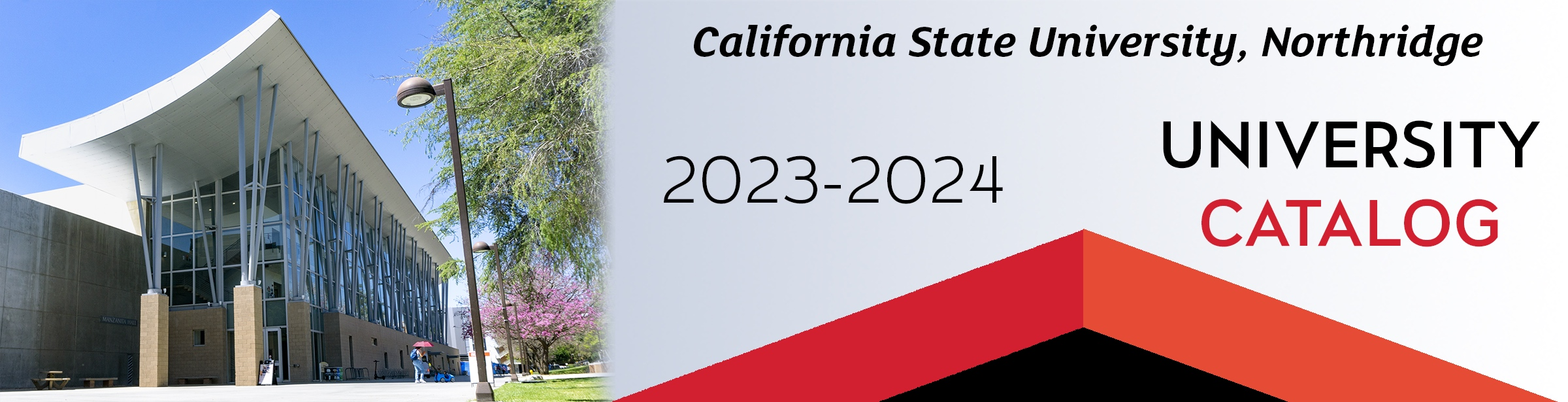## Program Description

The B.S. degree in Mathematics is designed for students who want to pursue occupational careers involving applied mathematics or want to prepare for graduate work in applied mathematics.

### Double Major

Students pursuing either a B.A. or a B.S. degree may combine a second major with Mathematics. In this circumstance, upon approval of an advisor, 6 units of upper division electives may be satisfied by courses in the second major. The remaining electives must be taken in the Department of Mathematics. Under certain rare conditions, the physics requirement in the lower division core may be replaced by appropriate coursework in the second major. Prior approval must be obtained from an advisor and the department chair for this latter occurrence.

## Program Requirements

In addition to University residence requirements for a bachelor’s degree, students must complete a minimum of 18 units of upper division Mathematics in residence at CSUN with the approval of a Mathematics advisor.

Students are assumed to have a facility in mathematics normally gained by recent completion of four years of high school mathematics through trigonometry and mathematical analysis. Because of the variation in curricula at the high school level, the Department of Mathematics offers the Mathematics Diagnostic Test (MDT) to ensure appropriate placement in the calculus sequence.

Students must complete the lower division core and one of the Mathematics B.S. options, and they must have at least a 2.0 GPA for all upper division units required in the major.

### 3. Upper Division Electives (15 units)

Students must take five elective courses chosen with an advisor from the following list, including at least one from MATH 450B and MATH 460.

MATH 366 Combinatorics (3)
MATH 440A Mathematical Statistics I (3)
MATH 440B Mathematical Statistics II (3)
MATH 450B Advanced Calculus II (3)
MATH 455 Complex Variables (3)
MATH 460 Abstract Algebra II (3)
MATH 463 Number Theory (3)
MATH 480 Partial Differential Equations (3)
MATH 481A Numerical Analysis (3)
MATH 481B Numerical Analysis (3)
MATH 481C Numerical Methods for Partial Differential Equations (3)
MATH 481D Topics in Numerical Mathematics (3)
MATH 483 Mathematical Modeling (3)
All 500-level Math courses, except those numbered MATH 510-514.

### 4. General Education (48 units)

Undergraduate students must complete 48 units of General Education as described in this Catalog, including 3 units of coursework meeting the Ethnic Studies (ES) graduation requirement.

12 units are satisfied by the following courses in the major: PHYS 220A satisfies B1 Physical Science; PHYS 220AL satisfies B3 Science Laboratory Activity; MATH 150A satisfies Basic Skills B4 Mathematics/Quantitative Reasoning; MATH 320 satisfies B5 Scientific Inquiry and Quantitative Reasoning; and COMP 110/L satisfies E Lifelong Learning.

Students are encouraged to take PHIL 230 to satisfy the Basic Skills A3 Critical Thinking requirement.

## Contact

Department of Mathematics
Chair: Katherine Stevenson
Live Oak Hall (LO) 1300
(818) 677-2721

## Program Learning Outcomes

Students receiving a Bachelor of Science in Mathematics will be able to:

1. Devise proofs of basic results concerning sets and number systems.
2. Rigorously establish fundamental analytic properties and results, such as limits, continuity, differentiability and integrability.
3. Demonstrate facility with the objects, terminology and concepts of linear algebra.
4. Demonstrate facility with the terminology, use of symbols and concepts of probability.
5. Write simple computer programs to perform computations arising in the mathematical sciences.